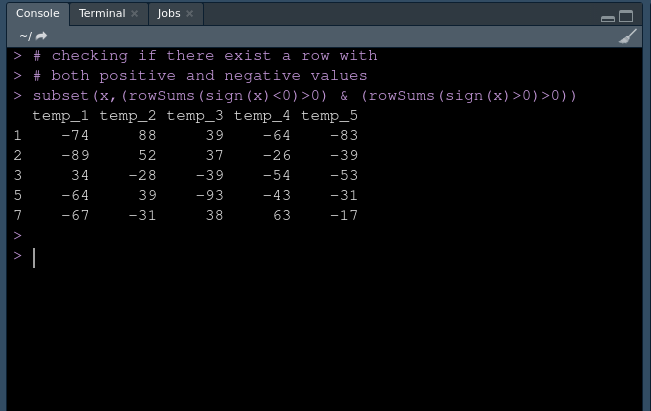GeeksforGeeks App
Open AppBrowser
Continue

# Select rows from R DataFrame that contain both positive and negative values

In this article, we will be discussing how to select rows in the data frame that contains both positive and negative values in R Programming Language. Let’s take an example for better understanding.

Suppose you have the following data frame in R that contains multiple columns and rows. All rows contain negative values or positive values or maybe both as follows.

Dataframe in use:

So, our task is to select only those rows which contain both positive and negative values. After selecting values, our data frame should look like this.

Expected output:

We are going to take a subset of the data frame if and only there is any row that contains values greater than 0 and less than 0, otherwise, we will not consider it.

Syntax:

subset(x,(rowSums(sign(x)<0)>0) & (rowSums(sign(x)>0)>0))

Here, x is the data frame name.

### Approach:

• Create dataset
• Apply subset()
• Select rows with both negative and positive values
• Display those rows

Example:

## R

 `# To select rows in dataframe ``# that contains both positive and negative values`` ` `# Creating dataset ``# creating fisrs column``first <- ``c``(-74,-89,34,-82,-64,92,-67)`` ` `# creating second column``second <- ``c``(88,52,-28,-19,39,86,-31)`` ` `# creating third column``third <- ``c``(39,37,-39,-64,-93,44,38)`` ` `# creating fourth column``fourth <- ``c``(-64,-26,-54,-33,-43,23,63)`` ` `# creating fifth column``fifth <- ``c``(-83,-39,-53,-20,-31,82,-17)`` ` `# creating dataframe``x <- ``data.frame``(temp_1=first,``                 ``temp_2=second,temp_3=third,``                 ``temp_4=fourth,temp_5=fifth)`` ` `# checking if there exist a row with ``# both positive and negative values``subset``(x,(``rowSums``(``sign``(x)<0)>0) & (``rowSums``(``sign``(x)>0)>0))`

Output:Figure 1

My Personal Notes arrow_drop_up